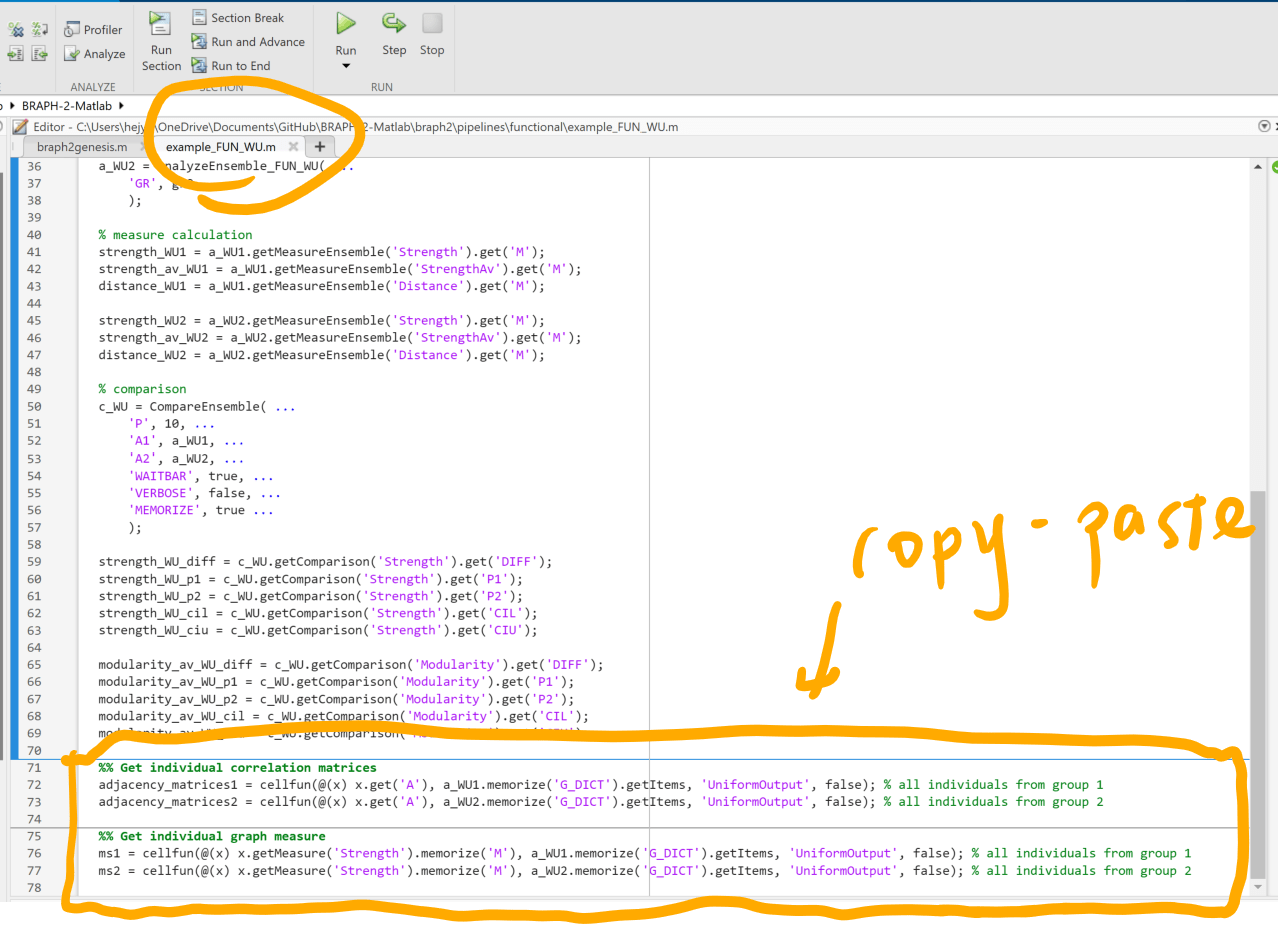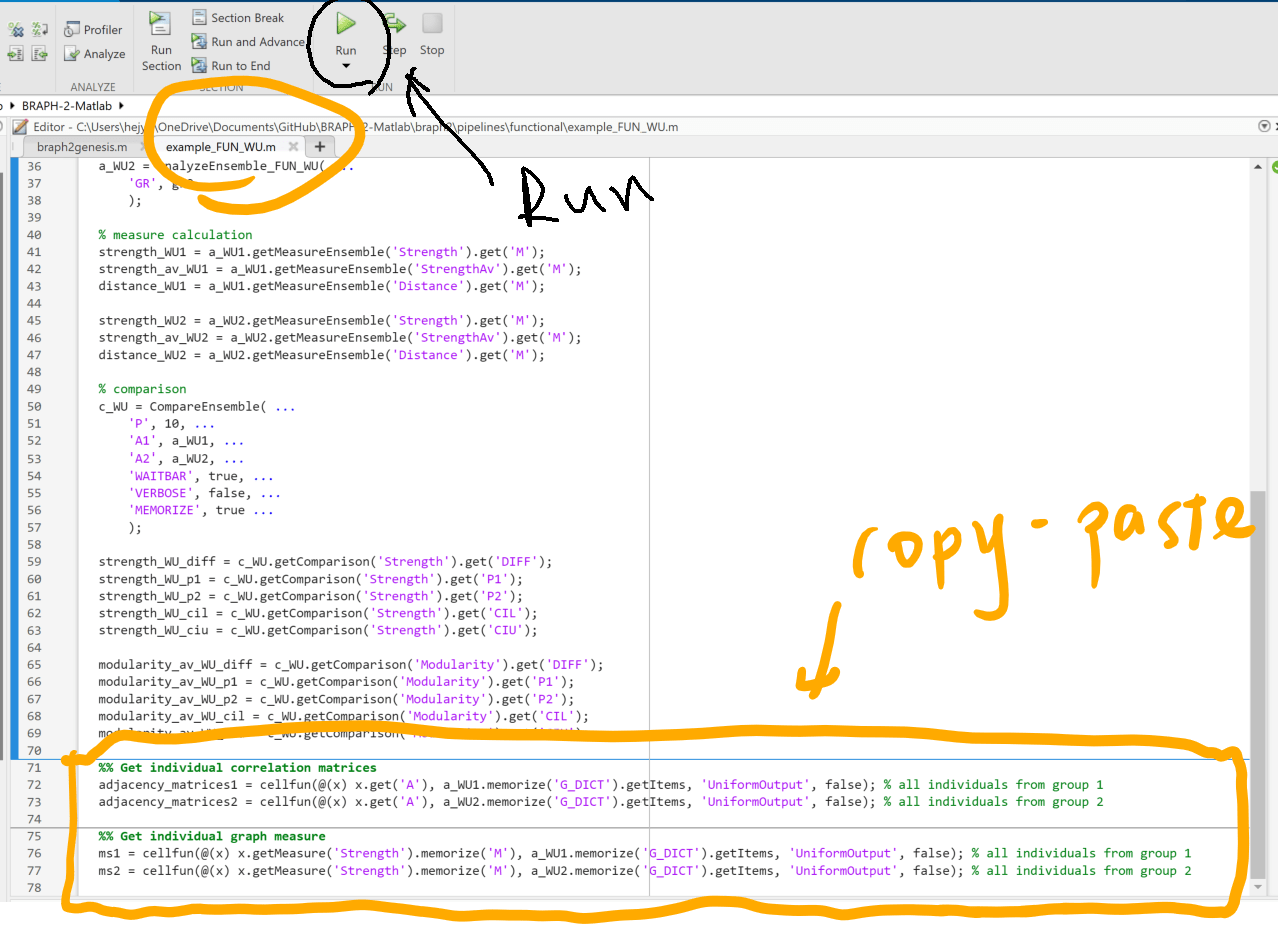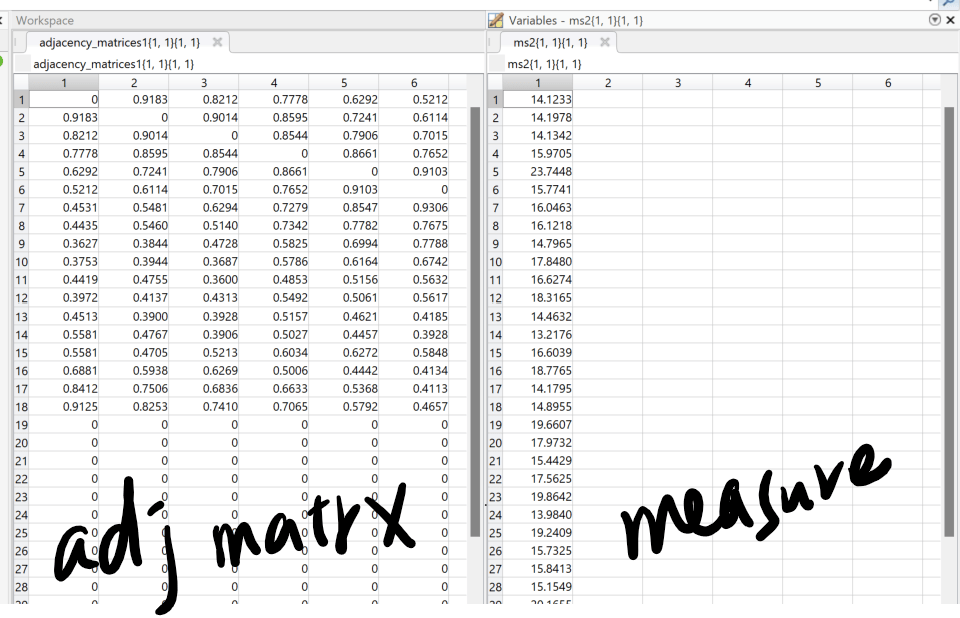# Export graph metric values

Home Forums General Help (Braph 2.0) Export graph metric values

Viewing 10 posts - 1 through 10 (of 15 total)
• Author
Posts
• #172555

Hi,
When I run the Functional analysis pipeline, is there anyway I can obtain the graph metrics values for individual subject and not just the group average. I need these values subject-wise and store them in a xls file.

Thanks.
Sai Prasanna

#173018

Dear Sai Prasanna,

The export to XLS file in the GUI is made on the group average results. Nevertheless, we keep the results for each subject. To obtain those, you will need to create your own script. Use ‘example_FUN_WU’ as a base, which is located in the Functional folder inside pipelines, and after calculating the analysis use the following line of code:

`ms1 = cellfun(@(x) x.getMeasure(measure_class).memorize('M'), analysis.memorize('G_DICT').getItems, 'UniformOutput', false);`

measure_class is the type of measure you want, for example, ‘Degree’.

Best regards,
Emiliano and Anna

#173060

Thanks. I will try that.

Also, the GUI is giving me an option of exporting the results as a JSON file only. Could you please let me know how I can save the group average results into an XLS file ?

#173076

I’m trying to use the example_FUN_BUD as you mentioned. I need to calculate all the graph metrics. The example file mentions 2-3 metrics. Do I use similar methods for all other metrics ?

I would appreciate your guidance on saving the individual subject correlation matrices as well.

#173528
1. You can export the results to XLS if you right-click on the top of the table that is visualized by pressing Data Selected Measures or Data Selected Comparison:Check Figure 11 and Figure 14 in this tutorial to see what I mean by going to Data Selected Measures or Data Selected Comparison: http://braph.org/tutorials/pipelines/pipeline-functional-comparison-bud/

2. You can add as many metrics as you want, just change the name of the Measure in the measure_class field. The example file is to show how to calculate them using a script and not the GUI. So you can modify it as you need.
3. To save the individual subject correlation matrices it is better to do it as well via script. Still, it is possible in the GUI if you press the IndexedDictionary button in the GRAPHS section in analysis.#173791

Sorry, if I’m asking too many questions.

1. I just tried as you mentioned. However, after running my script for calculating the analysis,

ms1 = cellfun(@(x) x.getMeasure(‘Degree’).memorize(‘M’), analysis.memorize(‘G_DICT’).getItems, ‘UniformOutput’, false)

gives me an error that says, “Unable to resolve the name analysis.memorize.”
I must be doing something wrong.

1. I have 200+ subjects. Saving individual correlation matrices through GUI would be a tedious process. How can I save them as separate .xls files for each subject through custom script?
#173810

Hi Sai Prasanna,

Thanks for the follow-up feedback.
I would like to provide further information based on example_FUN_WU.m about the following:
(1) Get individual graph metrics/graph measures.
(2) Get individual correlation matrices/adjacency matrices.

Step 1: Copy and paste the following codes at the end of example_FUN_WU.m

``````%% Get individual correlation matrices
adjacency_matrices1 = cellfun(@(x) x.get('A'), a_WU1.memorize('G_DICT').getItems, 'UniformOutput', false); % all individuals from group 1
adjacency_matrices2 = cellfun(@(x) x.get('A'), a_WU2.memorize('G_DICT').getItems, 'UniformOutput', false); % all individuals from group 2

%% Get individual graph measure
ms1 = cellfun(@(x) x.getMeasure('Strength').memorize('M'), a_WU1.memorize('G_DICT').getItems, 'UniformOutput', false); % all individuals from group 1
ms2 = cellfun(@(x) x.getMeasure('Strength').memorize('M'), a_WU2.memorize('G_DICT').getItems, 'UniformOutput', false); % all individuals from group 2``````Step 2: Run the revised example_FUN_WU.m by clicking on the run buttonStep 3: Check the variable adjacency_matrices1 for all individual correlation matrices (weighted-undirected in this example) from group 1, and adjacency_matrices2 for group 2

Step 4: Check the variable ms1 for all individual calculated metrics (strength in this example) from group 1, and ms2 for group 2Thank you, and let me know if you have any questions.
Best regards,
Yu-Wei

#178264

Thanks. That works perfectly for me.
On an average configured system and for two groups with around 400 subjects each, completing the above task takes about 65 hours. Is that a usual case?

#178289

Hi,

Thanks for the feedback.

May you provide further information:
what is the size of the adjacency matrix in your case? Additionally, I wanted to ask two things: (1) how long does it take to run the modified example file, example_FUN_WU.m, on your computer? and (2) have you installed the parallel computing toolbox?

For reference, on my computer with the parallel computing toolbox installed, each step in the modified example file example_FUN_WU.m took the following amount of time:
– Load Groups of SubjectFUN: 36 secs
– Measures calculation (Strength, StrengthAv, and Distance): 460 secs
– Comparison (Strength and modularity): 272 secs
– Get individual correlation matrices and graph measures: 1 sec

Therefore, if the comparison is not of your interest, you may skip the comparison calculation and save some time, which are the following lines:

``````% comparison
c_WU = CompareEnsemble( ...
'P', 10, ...
'A1', a_WU1, ...
'A2', a_WU2, ...
'WAITBAR', true, ...
'VERBOSE', false, ...
'MEMORIZE', true ...
);

strength_WU_diff = c_WU.getComparison('Strength').get('DIFF');
strength_WU_p1 = c_WU.getComparison('Strength').get('P1');
strength_WU_p2 = c_WU.getComparison('Strength').get('P2');
strength_WU_cil = c_WU.getComparison('Strength').get('CIL');
strength_WU_ciu = c_WU.getComparison('Strength').get('CIU');

modularity_av_WU_diff = c_WU.getComparison('Modularity').get('DIFF');
modularity_av_WU_p1 = c_WU.getComparison('Modularity').get('P1');
modularity_av_WU_p2 = c_WU.getComparison('Modularity').get('P2');
modularity_av_WU_cil = c_WU.getComparison('Modularity').get('CIL');
modularity_av_WU_ciu = c_WU.getComparison('Modularity').get('CIU');``````
#178296

I used AAL Atlas, and the size of adjacency matrices for each of the 800 subjects is 116 * 116.
Executing precisely the code you provided took approximately the same time, or maybe a little more. But not hours, for sure.
Yes, I have installed the parallel computing toolbox.
I did not execute the comparison part. I was interested in obtaining the correlation matrices of all the subjects and all the graph metric values for each subject (all those metrics listed on the GUI module for 800 odd subjects). This is what took approximately 65 hours. I just wanted to confirm with you if this is a reasonable time.

I appreciate your quick responses. It makes the toolbox extremely user-friendly.

Viewing 10 posts - 1 through 10 (of 15 total)
• You must be logged in to reply to this topic.